# A review of Dropout as applied to RNNs

In this post I will provide a background and overview of dropout and an analysis of dropout parameters as applied to language modelling using LSTM/GRU recurrent neural networks. After taking part 2 of the Deep Learning for Coders online course earlier this year I became intrigued by the application of RNNs to natural language processing. Core components of the fastai codebase were borrowed from the awd-lstm-lm project, and on delving into it’s code I wanted to better understand the regularisation strategies used.

In part 2 of this blog post I will show results of analysis on the effect and importance of dropout parameter variation on the resultant loss for RNNs used for language modelling and translation problems.

Dropout

Originally motivated by the role of sex in evolution, dropout was proposed by Hinton et al. (2012), whereby a unit in a neural network is temporarily removed from a network. Srivastava et al. (2014) applied dropout to feed forward neural network’s and RBM’s and noted a probability of dropout around 0.5 for hidden units and 0.2 for inputs worked well for a variety of tasks.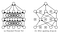Fig 1. After Srivastava et al. 2014. Dropout Neural Net Model. a) A standard neural net, with no dropout. b) Neural net with dropout applied.

The core concept of Srivastava el al. (2014) is that “each hidden unit in a neural network trained with dropout must learn to work with a randomly chosen sample of other units. This should make each hidden unit more robust and drive it towards creating useful features on its own without relying on other hidden units to correct its mistakes.”. “In a standard neural network, the derivative received by each parameter tells it how it should change so the final loss function is reduced, given what all other units are doing. Therefore, units may change in a way that they fix up the mistakes of the other units. This may lead to complex co-adaptations. This in turn leads to overfitting because these co-adaptations do not generalize to unseen data.” Srivastava et al. (2014) hypothesize that by making the presence of other hidden units unreliable, dropout prevents co-adaptation of each hidden unit.

For each training sample the network is re-adjusted and a new set of neurons are dropped out. At test time the weights are multiplied by their probability of their associated units’ dropout.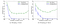Fig 2. After Srivastava el al., (2014). Effect of dropout rate on a) Constant number (n) of hidden units. b) Variable number of hidden units (n) multiplied by variable dropout probability (p) so that the number of hidden units after dropout (pn) is held constant.

We can see in Figure 2 a) that the test error is stable between around 0.4 and 0.8 probability of retaining a neuron (1-dropout). Test time errors increase as dropout is decreased below c. 0.2 (P>0.8), and with too much dropout (p<0.3) the network underfits.

Srivatava et al. (2104) further find that “as the size of the data set is increased, the gain from doing dropout increases up to a point and then declines. This suggests that for any given architecture and dropout rate, there is a “sweet spot” corresponding to some amount of data that is large enough to not be memorized in spite of the noise but not so large that overfitting is not a problem anyways.

Srivastava et al. (2014) multiplied hidden activations by Bernoulli distributed random variables which take the value 1 with probability p and 0 otherwise.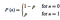Eq 1. Probability density function of a Bernoulli distribution of two outcomes — (in this case drop neuron or not) where probability of drop is given by p. The simpest example of a Bernoulli distribution is a coin toss, in which cas the probability (p) of heads is 0.5.

Source code for an example dropout layer is shown below.

`class Dropout():    def __init__(self, prob=0.5):        self.prob = prob        self.params = []    def forward(self,X):        self.mask = np.random.binomial(1,self.prob,size=X.shape) / self.prob        out = X * self.mask    return out.reshape(X.shape)    def backward(self,dout):        dX = dout * self.mask        return dX,[]`

Code 1: after deepnotes.io

DropConnect

Building further on Dropout, Wan et al. (2013) proposed DropConnect which “generalizes Dropout by randomly dropping the weights rather than the activations”. “With Drop connect each connection, rather than each output unit can be dropped with probability 1 − p” Wan et al. (2013). Like Dropout, the technique was only applied to fully connected layers.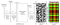Fig 3. After Wan et al. (2013) (a): An example model layout for a single DropConnect layer. After running feature extractor g() on input x, a random instantiation of the mask M (e.g. (b)), masks out the weight matrix W. The masked weights are multiplied with this feature vector to produce u which is the input to an activation function a and a softmax layer s. For comparison, c) shows an effective weight mask for elements that Dropout uses when applied to the previous layer’s output (columns) and this layer’s output (rows).

How DropConnect differers from Droput can be visualised when we see the basic structure of a neuron in neural net, as per the figure below. By appling dropout to input weights rather than the activations, DropConnect generalizes to the entire connectivity structure of a fully connected neural network layer.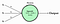Fig 4. after ml-cheatsheet.readthedocs.io. A neuron takes as an input a series of weights and applies a non-linear activation function to generate an output.

The two dropout methodologies mentioned above were applied to feed-foward convolutional neural networks. RNN’s differ from feed-forward only neural nets in that previous state is fed-back into the network, allowing the network to retain memory of previous states. As such, applying standard dropout to RNN’s tends limits the ability of the networks to retain their memory, hindering their performance. The issue with applying dropout to a recurrent neural network (RNN) was noted by Bayer et al. (2013) in that if the complete outgoing weight vecors were set to zero, the “resulting changes to the dynamics of an RNN during every forward pass are quite dramatic.”.

Example code to show how a RNN keeps this hidden state can bee seen in the code below from karpathy.github.io:

`class RNN:# ...def step(self, x):    # update the hidden state    self.h = np.tanh(np.dot(self.W_hh, self.h) + np.dot(self.W_xh, x))    # compute the output vector    y = np.dot(self.W_hy, self.h)    return yrnn = RNN()y = rnn.step(x) # x is an input vector, y is the RNN's output vector`

Dropout applied to RNN’s

As a way of overcoming performance issues with dropout applied to RNN’s, Zaremba et al. (2014) and Pham et al. (2013) applied dropout only to the non-recurrent connections (Dropout was not applied to the hidden states). “By not using dropout on the recurrent connections, the LSTM can benefit from dropout regularization without sacrificing its valuable memorization ability.” (Zaremba et al.,2014)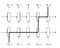Fig 8. after Zaremba et al. (2014) Regularized multilayer RNN. Dropout is only applied to the non-recurrent connections (ie only applied to the feedforward dashed lines). The thick line shows a typical path of information flow in the LSTM. The information is affected by dropout L + 1 times, where L is depth of network.

Variational Dropout

Gal and Ghahramani (2015) anaysed the application of dropout to the feedforward only parts of a RNN and found this approach still leads to overfitting. They proposed ‘variational dropout’ where by repeating “the same dropout mask at each time step for both inputs, outputs, and recurrent layers (drop the same network units at each time step)” using a bayesian interpretaton , they saw an improvement in Language Modelling and Sentiment Analysis tasks over ‘naive dropout’.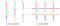Fig 9. after Gal and Ghahramani (2015). Naive dropout (a) (eg Zaremba et al., 2014) uses different masks at different time steps, with no dropout on the recurrent layers. Variational Dropout (b) uses the same dropout mask at each time step, including the recurrent layers (colours representing dropout masks, solid lines representing dropout, dashed lines representing standard connections with no dropout).

Recurrent Dropout’

Like Moon et al., (2015) and Gal and Ghahramani (2015), Semeniuta et al., (2016) proposed applying dropout to the recurrent connections of RNN’s so that recurrent weights could be regularized to improve performance. Gal and Ghahramani (2015) where use a network’s hidden state as input to sub-networks that compute gate values and cell updates and use dropout is to regularize the sub-networks (Fig. 9b below). Semeniuta et al., (2016) differers in that they consider “the architecture as a whole with the hidden state as its key part and regularize the whole network” (Fig. 9c below). This is similar to the concept of Moon et al., (2015) (as seen in Fig 9a below) but Semeniuta et al., (2016) found that dropping previous states directly as per Moon et al. (2015) produced mixed results and that applying dropout to the hidden state updates vector is a more principled way to drop recurrent connections.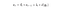Eg 1. after Semeniuta et al., (2016), where it, ft, are input and forget gates at step t; gt is the vector of cell updates and ct is the updated cell vector used to update the hidden state ht; and ∗ represent the element-wise multiplication. Dropout d is applied to the update vector gt.

“Our technique allows for adding a strong regularizer on the model weights responsible for learning short and long-term dependencies without affecting the ability to capture long- term relationships, which are especially important to model when dealing with natural language.” Semeniuta et al., (2016).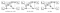Fig 10. after Semeniuta et al. “Illustration of the three types of dropout in recurrent connections of LSTM networks. Dashed arrows refer to dropped connections. Input connections are omitted for clarity.”. Note how Semeniuta et al. (2016) apply recurrent dropout to the updates to LSTM memory cells.

We demonstrate that recurrent dropout is most ef- fective when applied to hidden state update vec- tors in LSTMs rather than to hidden states; (ii) we observe an improvement in the network’s per- formance when our recurrent dropout is coupled with the standard forward dropout, though the extent of this improvement depends on the val- ues of dropout rates; (iii) contrary to our expec- tations, networks trained with per-step and per- sequence mask sampling produce similar results when using our recurrent dropout method, both being better than the dropout scheme proposed by Moon et al. (2015).” Semeniuta et al., (2016).

Zoneout

In a variation on the dropout philosophy, Krueger et al. (2017) proposed Zoneout where “instead of setting some units’ activations to 0 as in dropout, zoneout randomly replaces some units’ activations with their activations from the previous timestep.” this “makes it easier for the network to preserve information from previous timesteps going forward, and facilitates, rather than hinders, the flow of gradient information going backward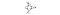Fig. 11 after Kruegar et al. (2017) Zoneout as a special case of dropout; ˜ht is the unit h’s hidden activation for the next time step (if not zoned out). Zoneout can be seen as applying dropout on the hidden state delta, ˜ht − ht−1. When this update is dropped out (represented by the dashed line), ht becomes ht−1.

While both recurrent dropout (Semeniuta et al., 2016) and Zoneout both prevent the loss of long-term memories built up in the states/cells of GRUs/LSTMS “zoneout does this by preserving units’ activations exactly. This difference is most salient when zoning out the hidden states (not the memory cells) of an LSTM, for which there is no analogue in recurrent dropout. Whereas saturated output gates or output nonlinearities would cause recurrent dropout to suffer from vanishing gradients (Bengio et al., 1994), zoned-out units still propagate gradients effectively in this situation. Furthermore, while the recurrent dropout method is specific to LSTMs and GRUs, zoneout generalizes to any model that sequentially builds distributed representations of its input, including vanilla RNNs.” Kruegar et al. (2017).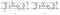Fig. 12. after Kruegar et al. (2017) (a) Zoneout, vs (b) the recurrent dropout strategy of (Semeniuta et al., 2016) in an LSTM. Dashed lines are zero-masked; in zoneout, the corresponding dotted lines are masked with the corresponding opposite zero-mask. Rectangular nodes are embedding layers.

The core concept of zoneout for tensorflow:

`if self.is_training:    new_state = (1 - state_part_zoneout_prob) * tf.python.nn_ops.dropout(                  new_state_part - state_part, (1 - state_part_zoneout_prob), seed=self._seed) + state_partelse:    new_state = state_part_zoneout_prob * state_part + (1 - state_part_zoneout_prob) * new_state_part`

AWD-LSTM

In a seminal work on regularization of RNNs for language modelling, Merity et al. (2017) proposed an approach they termed ASGD Weight-Dropped LSTM (AWD-LSTM). In this approach Merity et al., (2017) use DropConnect (Wan et al., 2013) on the recurrent hidden to hidden weight matrices, and variational dropout for all other dropout operations, as well as several other regularization strategies including randomized-length backpropagation through time (BPTT), activation regularization (AR), and temporal activation regularization (TAR).

Regarding the application of DropConnect Metity et al. 2017 mention “as the same weights are reused over multiple timesteps, the same individual dropped weights remain dropped for the entirety of the forward and backward pass. The result is similar to variational dropout, which applies the same dropout mask to recurrent connections within the LSTM by performing dropout on ht−1, except that the dropout is applied to the recurrent weights.”.

On the use of variational dropout Metity et al. 2017 note that “each example within the mini-batch uses a unique dropout mask, rather than a single dropout mask being used over all examples, ensuring diversity in the elements dropped out.”

By utilizing Embedding dropout like Gal & Ghahramani (2016), Metity et al. 2017 futher note that this “is equivalent to performing dropout on the embedding matrix at a word level, where the dropout is broadcast across all the word vector’s embedding.”. “As the dropout occurs on the embedding matrix that is used for a full forward and backward pass, this means that all occurrences of a specific word will disappear within that pass, equivalent to performing variational dropout on the connection between the one-hot embedding and the embedding”.

The code used by Merity et al. 2017 to apply variational dropout:

`class LockedDropout(nn.Module):    def __init__(self):        super().__init__()    def forward(self, x, dropout=0.5):        if not self.training or not dropout:            return x        m = x.data.new(1, x.size(1), x.size(2)).bernoulli_(1 - dropout)        mask = Variable(m, requires_grad=False) / (1 - dropout)        mask = mask.expand_as(x)        return mask * x`

where in the RNNModel(nn.Module) forward method dropout is applied thus (note self.lockdrop = LockedDropout(mask=mask)):

`def forward(self, input, hidden, return_h=False):    emb = embedded_dropout(self.encoder, input, dropout=self.dropoute if self.training else 0)    emb = self.lockdrop(emb, self.dropouti)    raw_output = emb    new_hidden = []    raw_outputs = []    outputs = []    for l, rnn in enumerate(self.rnns):        current_input = raw_output        raw_output, new_h = rnn(raw_output, hidden[l])        new_hidden.append(new_h)        raw_outputs.append(raw_output)        if l != self.nlayers - 1:            raw_output = self.lockdrop(raw_output, self.dropouth)            outputs.append(raw_output)    hidden = new_hidden    output = self.lockdrop(raw_output, self.dropout)    outputs.append(output)    result = output.view(output.size(0)*output.size(1), output.size(2))    if return_h:        return result, hidden, raw_outputs, outputs    return result, hidden`

DropConnect is applied in the __init__ method of the same RNNModel above thus:

`if rnn_type == 'LSTM':    self.rnns = [torch.nn.LSTM(ninp if l == 0 else nhid, nhid if l != nlayers - 1 else (ninp if tie_weights else nhid), 1, dropout=0) for l in range(nlayers)]    if wdrop:        self.rnns = [WeightDrop(rnn, ['weight_hh_l0'], dropout=wdrop) for rnn in self.rnns]`

With the key part of the WeightDrop class being the following method:

`def _setweights(self):    for name_w in self.weights:        raw_w = getattr(self.module, name_w + '_raw')        w = None        if self.variational:            mask = torch.autograd.Variable(torch.ones(raw_w.size(0), 1))            if raw_w.is_cuda: mask = mask.cuda()            mask = torch.nn.functional.dropout(mask, p=self.dropout, training=True)            w = mask.expand_as(raw_w) * raw_w        else:            w = torch.nn.functional.dropout(raw_w, p=self.dropout, training=self.training)        setattr(self.module, name_w, w)`

Fraternal Dropout

Resarch into dropout regularization has continued, with Zolna et al. 2017 proposing Fraternal Dropout. The methodology of Fraternal Dropout is to “minimize an equally weighted sum of prediction losses from two identical copies of the same LSTM with different dropout masks, and add as a regularization the L2 difference between the predictions (pre-softmax) of the two networks.”. Zolna et al. 2017 note that “the prediction of models with dropout generally vary with different dropout masks” and that ideally final predictions should be invariant to dropout masks. As such Fraternal Dropout attempts to minimize the variance in predictions under different dropout masks.

In fraternal dropout, we simultaneously feed-forward the input sample X through two identical copies of the RNN that share the same parameters θ but with different dropout masks sti and st j at each time step t. This yields two loss values at each time step t given by lt(pt(zt, sti; θ),Y), and lt(pt(zt, stj; θ),Y) as per the equation below:Eq 3 after Zolna et al. 2017. Overall loss function of Fraternal Dropout, where κ is the regularization coefficient, m is the dimensions of pt(zt, st i; θ) and RFD(zt; θ) is the fraternal dropout regularization.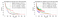Fig 13. after Zolna et al. 2017. Ablation study: Train (left) and validation (right) perplexity on PTB word level model- ing with single layer LSTM (10M parameters). These curves study the learning dynamics of the baseline model, temporal activity regularization (TAR), prediction regularization (PR), activity reg- ularization (AR) and fraternal dropout. Fraternal Dropout converges faster and generalizes better than the other regularizers in comparison.

Curriculum Dropout

Although currently only applied to feed forward Convolutional Neural Networks, Curriculum Droput proposed by Morerio et al., 2017 is an interesting line of research. Morerio et al. 2017 propose a “scheduling for dropout training applied to deep neural networks. By softly increasing the amount of units to be suppressed layerwise, we achieve an adaptive regularization and provide a better smooth initialization for weight optimization.”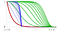Fig 14. after Morerio et al., 2017. Curriculum functions for dropout, where dropout is increased with time based on various functions.

References:

J. Bayer, C. Osendorfer, D. Korhammer, N. Chen, S. Urban, P. van der Smagt. 2013. On Fast Dropout and its Applicability to Recurrent Networks.

Y. Bengio, P. Simard, P. Frasconi. 1994. Learning long-term dependencies with gradient descent is difficult.

deepnotes.io. https://deepnotes.io/dropout

Y. Gal, abd Z. Ghahramani. 2015. A Theoretically Grounded Application of Dropout in Recurrent Neural Networks.

G. E. Hinton, N. Srivastava, A. Krizhevsky, I. Sutskever and R. R. Salakhutdinov. 2012. Improving neural networks by preventing co-adaptation of feature detectors.

karpathy.github.io. https://karpathy.github.io/2015/05/21/rnn-effectiveness/

D. Krueger, T. Maharaj, J. Kramár, M. Pezeshki, N. Ballas, N. Rosemary Ke, A. Goyal, Y. Bengio, A. Courville, C. Pal. 2016. Zoneout: Regularizing RNNs by Randomly Preserving Hidden Activations.

S. Merity, N. Shirish Keskar, R. Socher. 2017. Regularizing and Optimizing LSTM Language Models.

T. Moon, H. Choi, H. Lee, I. Song. 2015. Rnndrop: A novel dropout for rnns.

P. Morerio, J. Cavazza, R. Volpi, R.Vidal, V. Murino. 2017. Curriculum Dropout

A. Narwekar, A. Pampari. 2016. Recurrent Neural Network Architectures. http://slazebni.cs.illinois.edu/spring17/lec20_rnn.pdf

V. Pham, T. Bluche, C. Kermorvant, J. Louradour. 2013. Dropout improves Recurrent Neural Networks for Handwriting Recognition

S. Semeniuta, A. Severyn, E. Barth. 2016. Recurrent Dropout without Memory Loss.

N. Srivastava, G. Hinton, A. Krizhevsky, I. Sutskever, R. Salakhutdinov. 2014. Dropout: A Simple Way to Prevent Neural Networks from Overfitting.

L. Wan, M. Zeiler, Matthew, S. Zhang, Y. LeCun, R. Fergus. 2013. Regularization of neural networks using dropconnect.

W. Zaremba, I. Sutskever, O. Vinyals. 2014. Recurrent Neural Network Regularization

K. Zolna, D. Arpit, D. Suhubdy, Y. Bengio. 2017. Fraternal Dropout

Geophysicist and Deep Learning Practitioner